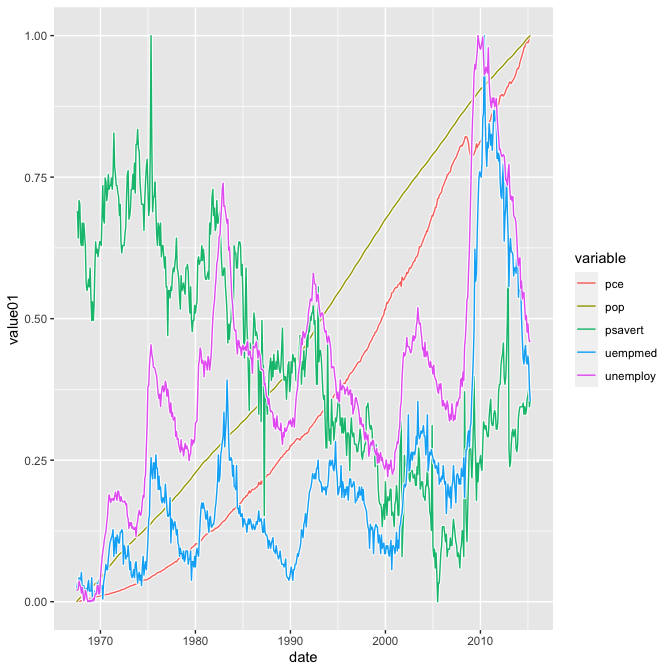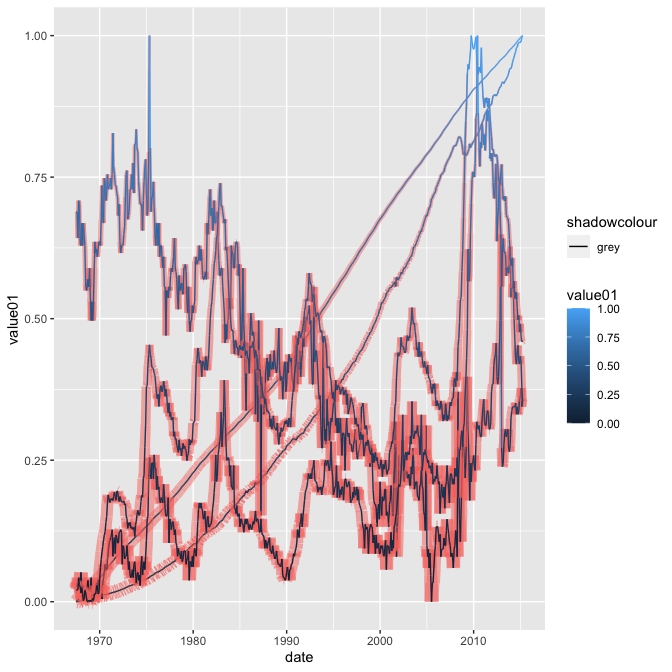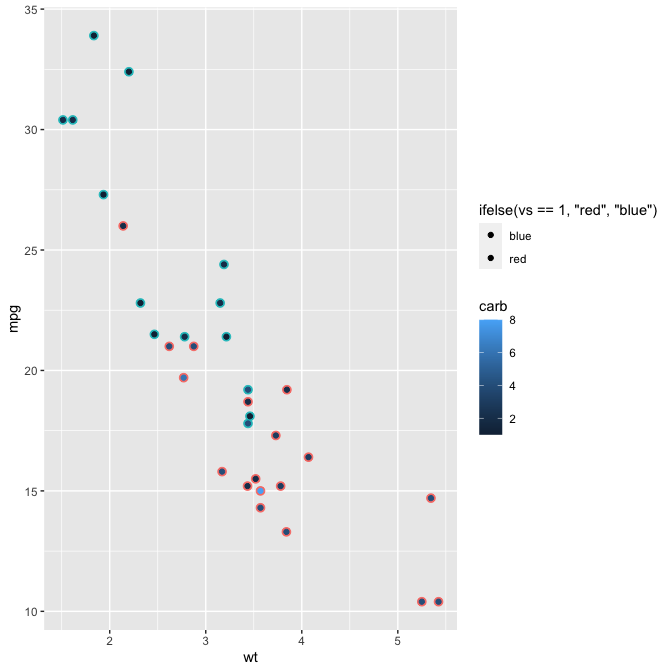# Shadow path, line and step

## shadow line: geom_shadowline()

The most simple use of geom_shadowline() is a simple replacement of geom_line()

library(ggshadow)

ggplot(economics_long, aes(date, value01, colour = variable)) + geom_shadowline()## varying line color

It is possible to draw lines with varying colors, and it is also possible to change the color, alpha and size of the shadow using shadowcolor, shadowsize and shadowalpha.

library(ggshadow)

ggplot(economics_long, aes(date, value01, group = variable, colour=value01, shadowcolor='grey', shadowalpha=0.5, shadowsize=5*(1-value01))) + geom_shadowline()## Shadow point example: geom_shadowpoint()

Example using geom_shadowpoint().

ggplot(mtcars, aes(wt, mpg)) + geom_shadowpoint(aes( color = carb, shadowcolour = ifelse(vs == 1, 'red', 'blue') ))